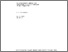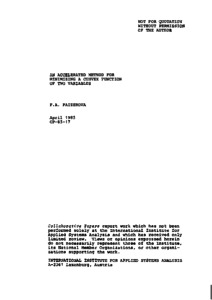# An Accelerated Method for Minimizing a Convex Function of Two Variables

Paizerova, F.A. (1985). An Accelerated Method for Minimizing a Convex Function of Two Variables. IIASA Collaborative Paper. IIASA, Laxenburg, Austria: CP-85-017Preview
Text
CP-85-017.pdf

## Abstract

The author considers the problem of minimizing a convex function of two variables without computing the derivatives or (in the nondifferentiable case) the subgradients of the function, and suggests two algorithms for doing this. Such algorithms could form an integral part of new methods for minimizing a convex function of many variables based on the solution of a two-dimensional minimization problem at each step (rather than on line-searches, as in most existing algorithms).

This is a contribution to research on nonsmooth optimization currently underway in System and Decision Sciences Program Core.

Item Type: Monograph (IIASA Collaborative Paper) System and Decision Sciences - Core (SDS) IIASA Import 15 Jan 2016 01:56 27 Aug 2021 17:12 http://pure.iiasa.ac.at/2731View Item